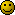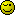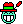# Funny javascript

edited December 2010
You wanna have some fun ?Go to any website that use images and replace the url in your browser with this javascript :
```javascript:R=0;x1=.1;y1=.05;x2=.25;y2=.24;x3=1.6;y3=.24;x4=300;y4=200;x5=300;y5=200;DI=document.getElementsByTagName("img");DIL=DI.length;function%20A(){for(i=0;i-DIL;i++){DIS=DI[i].style;DIS.position='absolute';DIS.left=(Math.sin(R*x1+i*x2+x3)*x4+x5)+"px";DIS.top=(Math.cos(R*y1+i*y2+y3)*y4+y5)+"px"}R++}setInterval('A()',50);void(0);
```

It makes an animation with every image found on the page :P

I now share this little "eye candy" with some carefully written javascript with all of you IC members hahIt's funny what you can do with JS, even on website that are not yours to play with :P

Hahahah